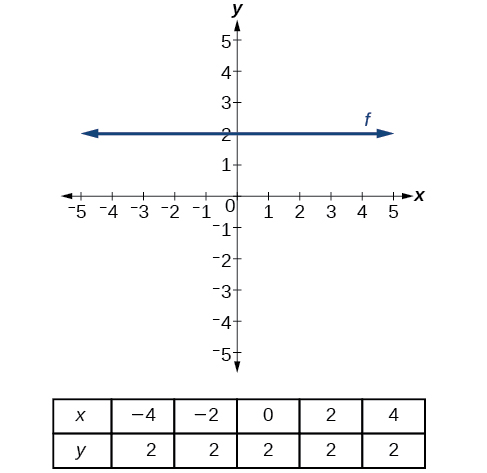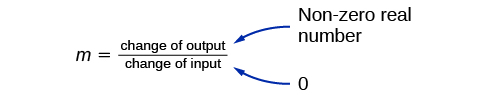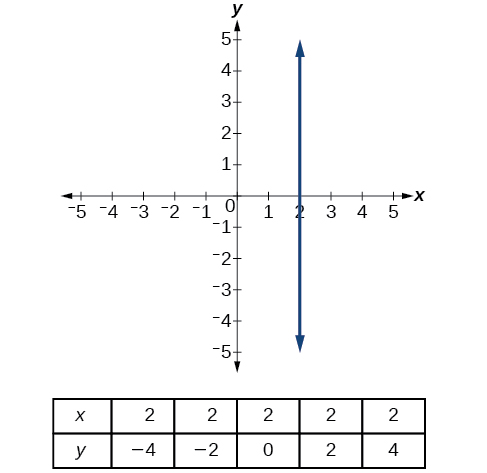# 4.1 Linear functions  (Page 11/27)

 Page 11 / 27A horizontal line representing the function   f ( x ) = 2

A vertical line    indicates a constant input, or x -value. We can see that the input value for every point on the line is 2, but the output value varies. Because this input value is mapped to more than one output value, a vertical line does not represent a function. Notice that between any two points, the change in the input values is zero. In the slope formula, the denominator will be zero, so the slope of a vertical line is undefined.Example of how a line has a vertical slope. 0 in the denominator of the slope.

A vertical line, such as the one in [link] , has an x -intercept, but no y- intercept unless it’s the line $\text{\hspace{0.17em}}x=0.\text{\hspace{0.17em}}$ This graph represents the line $\text{\hspace{0.17em}}x=2.$The vertical line,   x = 2 ,   which does not represent a function

## Horizontal and vertical lines

Lines can be horizontal or vertical.

A horizontal line    is a line defined by an equation in the form $\text{\hspace{0.17em}}f\left(x\right)=b.$

A vertical line    is a line defined by an equation in the form $\text{\hspace{0.17em}}x=a.$

## Writing the equation of a horizontal line

Write the equation of the line graphed in [link] .

For any x -value, the y -value is $\text{\hspace{0.17em}}-4,\text{\hspace{0.17em}}$ so the equation is $\text{\hspace{0.17em}}y=-4.$

## Writing the equation of a vertical line

Write the equation of the line graphed in [link] .

The constant x -value is $\text{\hspace{0.17em}}7,$ so the equation is $\text{\hspace{0.17em}}x=7.$

## Determining whether lines are parallel or perpendicular

The two lines in [link] are parallel lines    : they will never intersect. They have exactly the same steepness, which means their slopes are identical. The only difference between the two lines is the y -intercept. If we shifted one line vertically toward the other, they would become coincident.

We can determine from their equations whether two lines are parallel by comparing their slopes. If the slopes are the same and the y -intercepts are different, the lines are parallel. If the slopes are different, the lines are not parallel.

Unlike parallel lines, perpendicular lines    do intersect. Their intersection forms a right, or 90-degree, angle. The two lines in [link] are perpendicular.

Perpendicular lines do not have the same slope. The slopes of perpendicular lines are different from one another in a specific way. The slope of one line is the negative reciprocal of the slope of the other line. The product of a number and its reciprocal is $\text{\hspace{0.17em}}1.\text{\hspace{0.17em}}$ So, if are negative reciprocals of one another, they can be multiplied together to yield $\text{\hspace{0.17em}}–1.$

${m}_{1}{m}_{2}=-1$

To find the reciprocal of a number, divide 1 by the number. So the reciprocal of 8 is $\text{\hspace{0.17em}}\frac{1}{8},\text{\hspace{0.17em}}$ and the reciprocal of $\text{\hspace{0.17em}}\frac{1}{8}\text{\hspace{0.17em}}$ is 8. To find the negative reciprocal, first find the reciprocal and then change the sign.

As with parallel lines, we can determine whether two lines are perpendicular by comparing their slopes, assuming that the lines are neither horizontal nor vertical. The slope of each line below is the negative reciprocal of the other so the lines are perpendicular.

The product of the slopes is –1.

#### Questions & Answers

write down the polynomial function with root 1/3,2,-3 with solution
if A and B are subspaces of V prove that (A+B)/B=A/(A-B)
write down the value of each of the following in surd form a)cos(-65°) b)sin(-180°)c)tan(225°)d)tan(135°)
Prove that (sinA/1-cosA - 1-cosA/sinA) (cosA/1-sinA - 1-sinA/cosA) = 4
what is the answer to dividing negative index
In a triangle ABC prove that. (b+c)cosA+(c+a)cosB+(a+b)cisC=a+b+c.
give me the waec 2019 questions
the polar co-ordinate of the point (-1, -1)
prove the identites sin x ( 1+ tan x )+ cos x ( 1+ cot x )= sec x + cosec x
tanh`(x-iy) =A+iB, find A and B
B=Ai-itan(hx-hiy)
Rukmini
what is the addition of 101011 with 101010
If those numbers are binary, it's 1010101. If they are base 10, it's 202021.
Jack
extra power 4 minus 5 x cube + 7 x square minus 5 x + 1 equal to zero
the gradient function of a curve is 2x+4 and the curve passes through point (1,4) find the equation of the curve
1+cos²A/cos²A=2cosec²A-1
test for convergence the series 1+x/2+2!/9x3ByByBy Gerr ZenBy RhodesBy